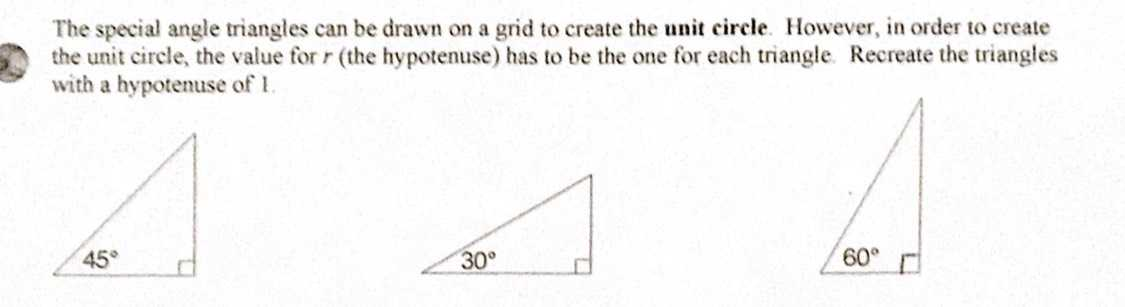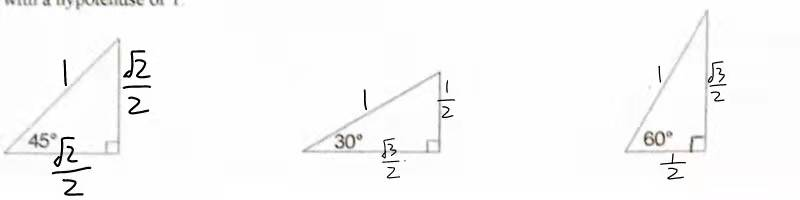### Still have math questions?The special angle triangles can be drawn on a grid to create the unit circle. However, in order to create the unit circle, the value for $$r$$ (the hypotenuse) has to be the one for each triangle. Recreate the triangles with a hypotenuse of $$1$$ .